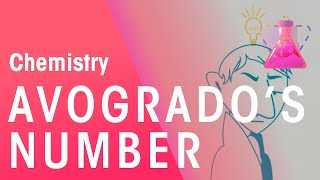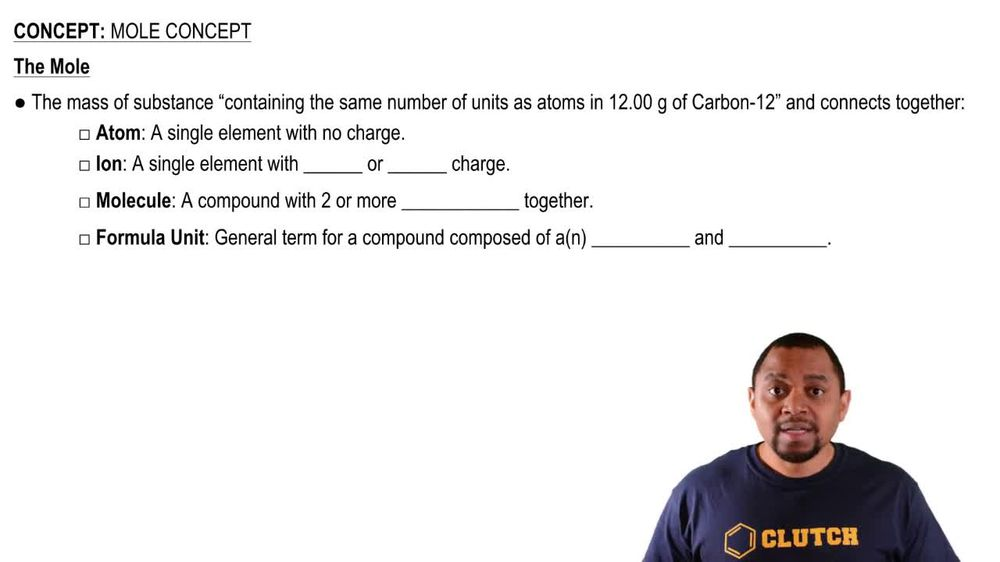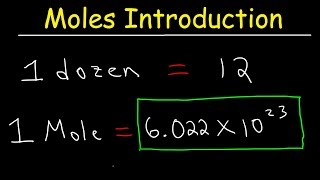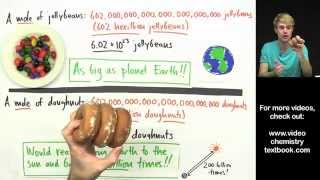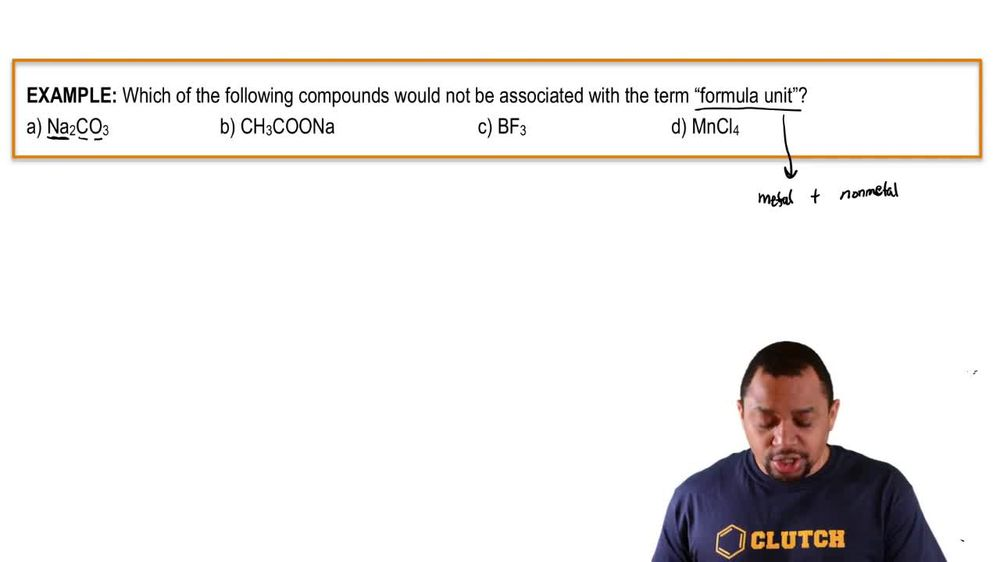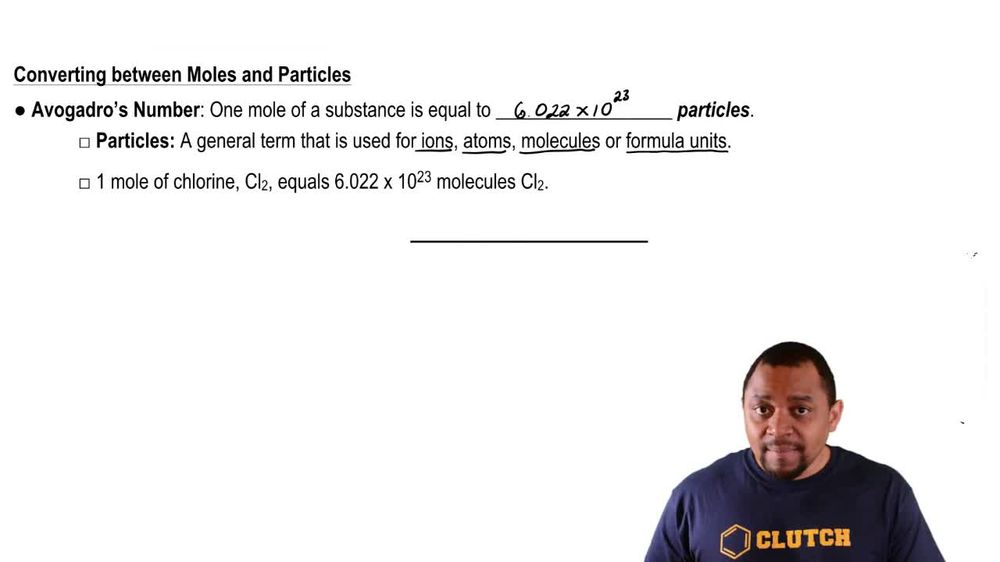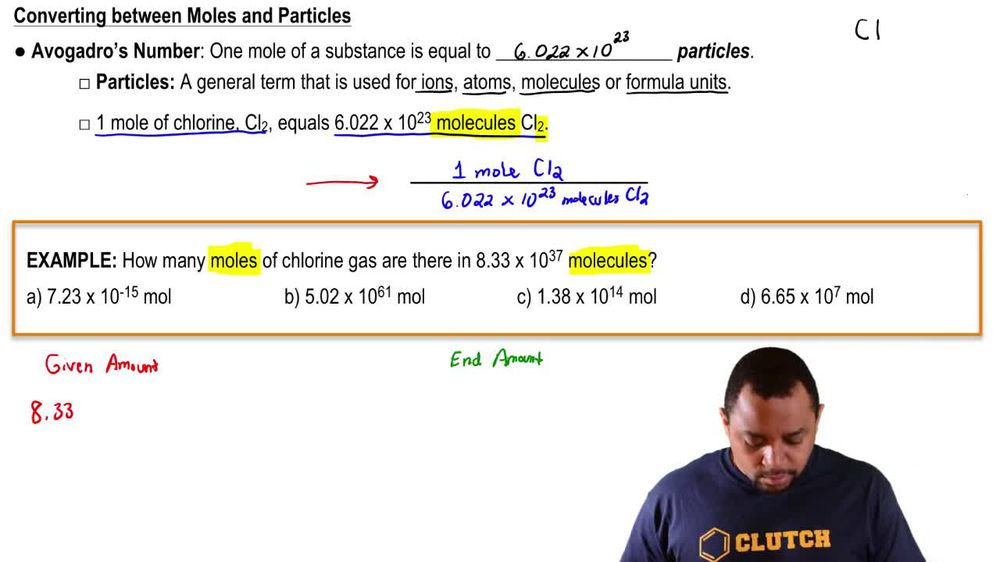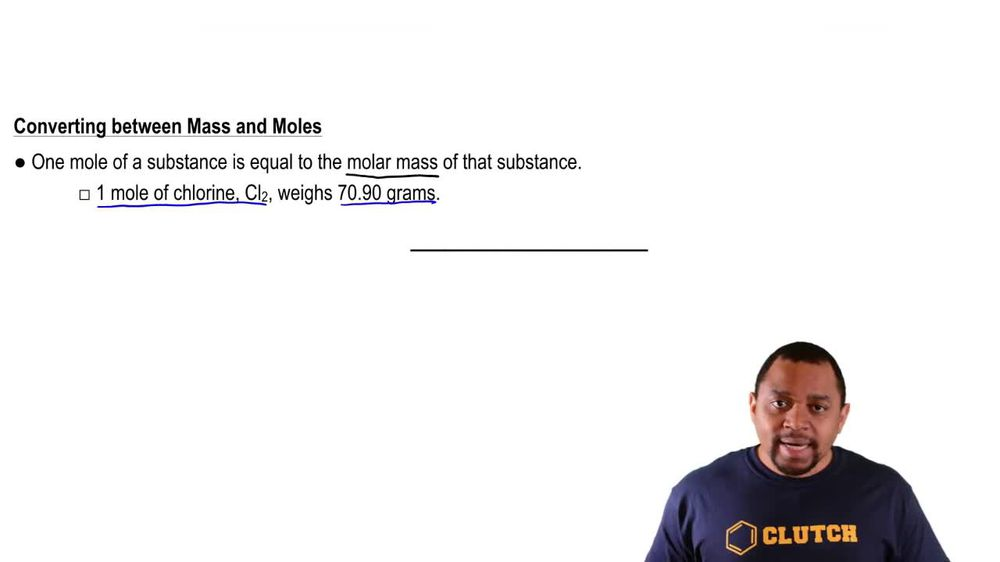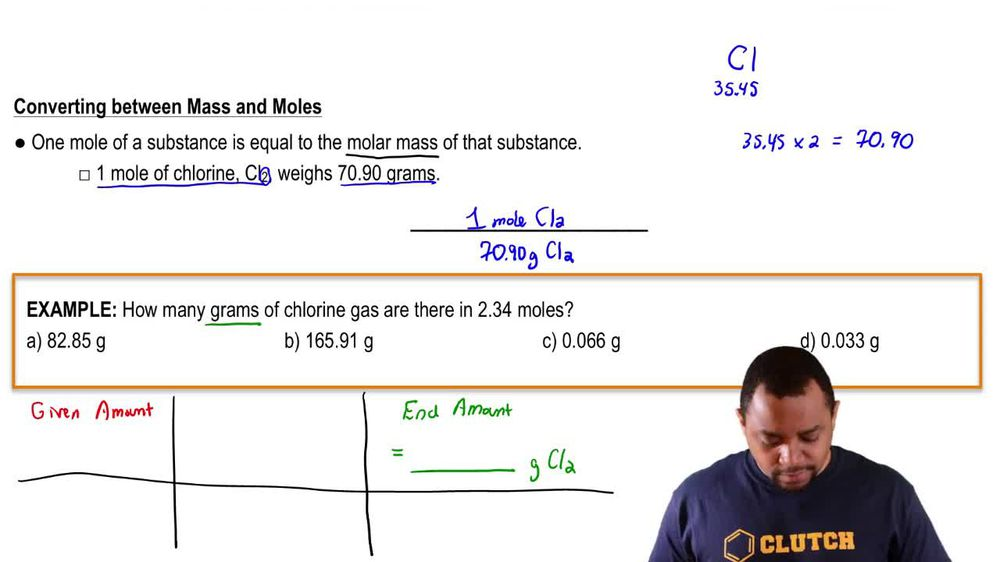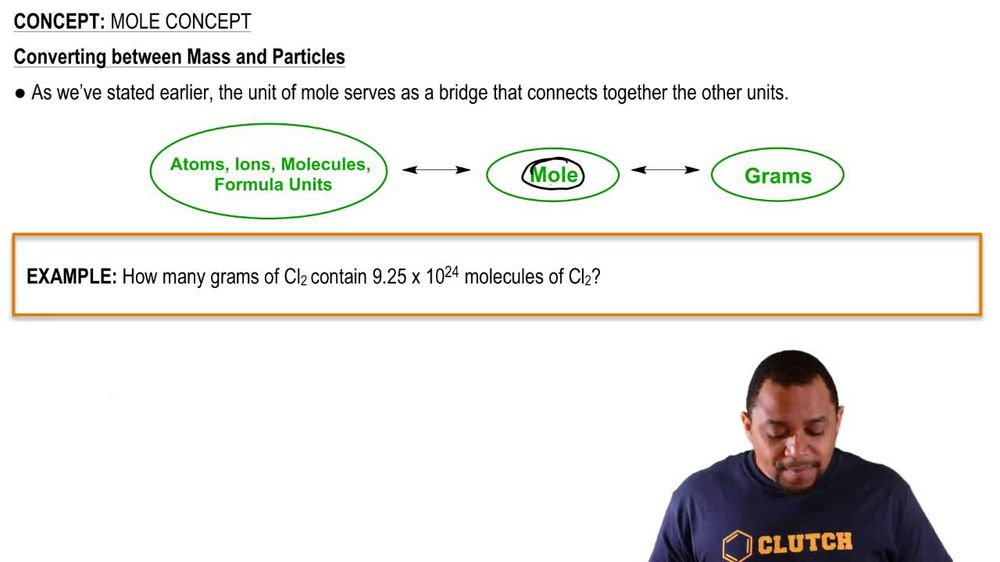Start typing, then use the up and down arrows to select an option from the list.
1. 2. Atoms & Elements2. Mole Concept
Problem

# What is the mass, in kilograms, of an Avogadro’s number of people, if the average mass of a person is 160 lb?

Relevant Solution1m
Play a video:
Hey everyone where as to calculate the mass in kg of three Alva Gaudreau numbers of apples. If each apple weighs 0.3, three lbs on average. To answer this question, we need to know the relationship between pounds and kilograms and as we've learned, one kg is equivalent to £2.2. Now, let's answer this question. We were told that we had three avocado numbers of apples And we know avocados number is 6.02, 2 times 10 to the 23rd, and each apple weighs £0.33. So we're going to multiply this value by £0.33. And now we're going to use our dimensional analysis and convert our pounds into kilogram. And as we stated earlier, we know that £2.. equivalent to one kg. So when we calculate this out and cancel out our unit, We end up with a value of 2.7 times 10 to the 23rd kg. And this is going to be our final answer. Now, I hope this made sense. And let us know if you have any questions